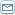# Learning To Trade Forex - Calculating Pip Values

 By: Donald SaundersUnderstanding pips is extremely important as a pip denotes the smallest movement in the price of a currency and it is this movement which determines your profit or loss when closing your trading position.For most, but not all, currencies a pip is 0.0001 or 0.01%. So, when a currency moves from a price of 1.7800 to 1.7805 it is said to move 5 pips.Perhaps the easiest way to understand how to calculate pip values is to start by looking at currency pairs involving the US Dollar. In any quote the US Dollar can be either the base currency or the counter or quote currency and we'll start by considering the situation when the US Dollar is the quote currency as in the case of EUR/USD, CAD/USD or GBP/USD.In this case the situation is simple because a pip will always have a value of \$10. So, if you are trading GBP/USD and the market moves in your favor by 5 pips you will make a profit of \$50. Let's see this in action.Supposing the rate for GBP/USD is 1.9340. This means that 1 UK Pound is worth 1.9340 US Dollars. Bearing in mind that a standard interbank lot size is 100,000 this means that 100,000 UK Pounds are worth 193,400 US Dollars. If the market now moves 1 pip so that GBP/USD is 1.9341 then 100,000 UK Pounds will now be worth 193,410 US Dollars - a rise of \$10.Now let's look at the situation when the US Dollar is the base currency and consider a quote of USD/GBP = 0.5170. Here 1 US Dollar is worth 0.5170 UK Pounds and 100,000 US Dollars are worth 517,000 UK Pounds.If the price moves up 1 pip then USD/GBP = 0.5171 and 1 US Dollar is worth 0.5171 UK Pounds and 100,000 US Dollars is worth 517,100 UK Pounds.In this case a movement of 1 pip represents a value of 100 UK Pounds which, translated into US Dollars gives a pip value of 193.39 US Dollars (100 ÷ 0.5171).From this we can see that with the US Dollar as the quote currency a pip will have a value of \$10 for a standard trading lot but that the pip value will vary with the market price when the US Dollar is the base currency.